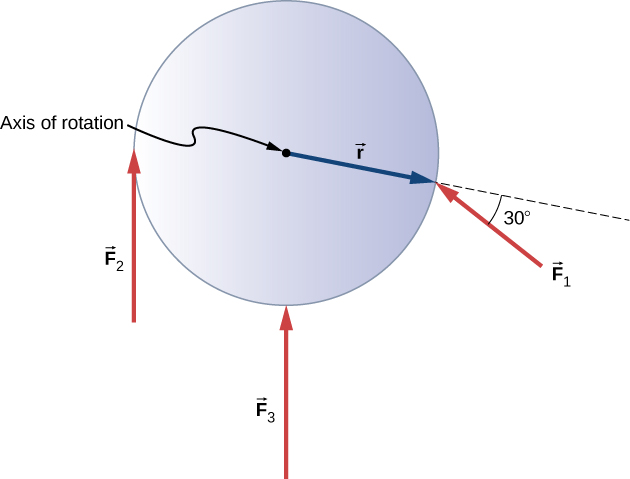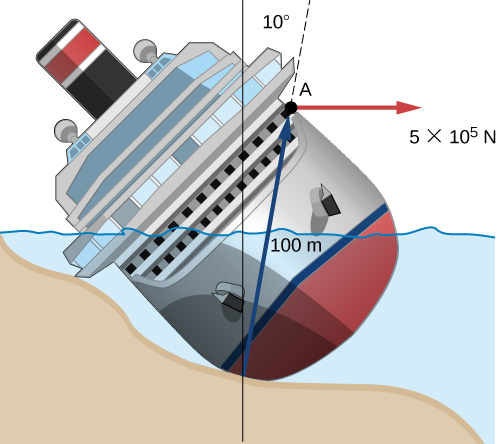# 10.6 Torque  (Page 3/6)

 Page 3 / 6

## Calculating torque on a rigid body

[link] shows several forces acting at different locations and angles on a flywheel. We have $|{\stackrel{\to }{F}}_{1}|=20\phantom{\rule{0.2em}{0ex}}\text{N},$ $|{\stackrel{\to }{F}}_{2}|=30\phantom{\rule{0.2em}{0ex}}\text{N}$ , $|{\stackrel{\to }{F}}_{3}|=30\phantom{\rule{0.2em}{0ex}}\text{N}$ , and $r=0.5\phantom{\rule{0.2em}{0ex}}\text{m}$ . Find the net torque on the flywheel about an axis through the center.Three forces acting on a flywheel.

## Strategy

We calculate each torque individually, using the cross product, and determine the sign of the torque. Then we sum the torques to find the net torque.

## Solution

We start with ${\stackrel{\to }{F}}_{1}$ . If we look at [link] , we see that ${\stackrel{\to }{F}}_{1}$ makes an angle of $90\text{°}+60\text{°}$ with the radius vector $\stackrel{\to }{r}$ . Taking the cross product, we see that it is out of the page and so is positive. We also see this from calculating its magnitude:

$|{\stackrel{\to }{\tau }}_{1}|=r{F}_{1}\text{sin}\phantom{\rule{0.2em}{0ex}}150\text{°}=0.5\phantom{\rule{0.2em}{0ex}}\text{m}\left(20\phantom{\rule{0.2em}{0ex}}\text{N}\right)\left(0.5\right)=5.0\phantom{\rule{0.2em}{0ex}}\text{N}·\text{m}.$

Next we look at ${\stackrel{\to }{F}}_{2}$ . The angle between ${\stackrel{\to }{F}}_{2}$ and $\stackrel{\to }{r}$ is $90\text{°}$ and the cross product is into the page so the torque is negative. Its value is

$|{\stackrel{\to }{\tau }}_{2}|=\text{−}r{F}_{2}\text{sin}\phantom{\rule{0.2em}{0ex}}90\text{°}=-0.5\phantom{\rule{0.2em}{0ex}}\text{m}\left(30\phantom{\rule{0.2em}{0ex}}\text{N}\right)=-15.0\phantom{\rule{0.2em}{0ex}}\text{N}·\text{m}.$

When we evaluate the torque due to ${\stackrel{\to }{F}}_{3}$ , we see that the angle it makes with $\stackrel{\to }{r}$ is zero so $\stackrel{\to }{r}\phantom{\rule{0.2em}{0ex}}×\phantom{\rule{0.2em}{0ex}}{\stackrel{\to }{F}}_{3}=0.$ Therefore, ${\stackrel{\to }{F}}_{3}$ does not produce any torque on the flywheel.

We evaluate the sum of the torques:

${\tau }_{\text{net}}=\sum _{i}|{\tau }_{i}|=5-15=-10\phantom{\rule{0.2em}{0ex}}\text{N}·\text{m}.$

## Significance

The axis of rotation is at the center of mass of the flywheel. Since the flywheel is on a fixed axis, it is not free to translate. If it were on a frictionless surface and not fixed in place, ${\stackrel{\to }{F}}_{3}$ would cause the flywheel to translate, as well as ${\stackrel{\to }{F}}_{1}$ . Its motion would be a combination of translation and rotation.

Check Your Understanding A large ocean-going ship runs aground near the coastline, similar to the fate of the Costa Concordia , and lies at an angle as shown below. Salvage crews must apply a torque to right the ship in order to float the vessel for transport. A force of $5.0\phantom{\rule{0.2em}{0ex}}×\phantom{\rule{0.2em}{0ex}}{10}^{5}\phantom{\rule{0.2em}{0ex}}\text{N}$ acting at point A must be applied to right the ship. What is the torque about the point of contact of the ship with the ground ( [link] )?A ship runs aground and tilts, requiring torque to be applied to return the vessel to an upright position.

The angle between the lever arm and the force vector is $80\text{°};$ therefore, ${r}_{\perp }=100\text{m(sin80}\text{°}\right)=98.5\phantom{\rule{0.2em}{0ex}}\text{m}$ .

The cross product $\stackrel{\to }{\tau }=\stackrel{\to }{r}\phantom{\rule{0.2em}{0ex}}×\phantom{\rule{0.2em}{0ex}}\stackrel{\to }{F}$ gives a negative or clockwise torque.

The torque is then $\tau =\text{−}{r}_{\perp }F=-98.5\phantom{\rule{0.2em}{0ex}}\text{m}\left(5.0\phantom{\rule{0.2em}{0ex}}×\phantom{\rule{0.2em}{0ex}}{10}^{5}\text{N}\right)=-4.9\phantom{\rule{0.2em}{0ex}}×\phantom{\rule{0.2em}{0ex}}{10}^{7}\text{N}·\text{m}$ .

## Summary

• The magnitude of a torque about a fixed axis is calculated by finding the lever arm to the point where the force is applied and using the relation $|\stackrel{\to }{\tau }|={r}_{\perp }F$ , where ${r}_{\perp }$ is the perpendicular distance from the axis to the line upon which the force vector lies.
• The sign of the torque is found using the right hand rule. If the page is the plane containing $\stackrel{\to }{r}$ and $\stackrel{\to }{F}$ , then $\stackrel{\to }{r}\phantom{\rule{0.2em}{0ex}}×\phantom{\rule{0.2em}{0ex}}\stackrel{\to }{F}$ is out of the page for positive torques and into the page for negative torques.
• The net torque can be found from summing the individual torques about a given axis.

## Conceptual questions

What three factors affect the torque created by a force relative to a specific pivot point?

magnitude of the force, length of the lever arm, and angle of the lever arm and force vector

Give an example in which a small force exerts a large torque. Give another example in which a large force exerts a small torque.

When reducing the mass of a racing bike, the greatest benefit is realized from reducing the mass of the tires and wheel rims. Why does this allow a racer to achieve greater accelerations than would an identical reduction in the mass of the bicycle’s frame?

The moment of inertia of the wheels is reduced, so a smaller torque is needed to accelerate them.

#### Questions & Answers

when I click on the links in the topics noting shows. what should I do.
can we regard torque as a force?
Torque is only referred a force to rotate objects.
SHREESH
thanks
Emmanuel
I need lessons on Simple harmonic motion
Emmanuel
what is the formulae for elastic modulus
Given two vectors, vector C which is 3 units, and vector D which is 5 units. If the two vectors form an angle of 45o, determine C D and direction.
At time to = 0 the current to the DC motor is reverse, resulting in angular displacement of the motor shafts given by angle = (198rad/s)t - (24rad/s^2)t^2 - (2rad/s^3)t^3 At what time is the angular velocity of the motor shaft zero
3s
Basit
what is angular velocity
In three experiments, three different horizontal forces are ap- plied to the same block lying on the same countertop. The force magnitudes are F1 " 12 N, F2 " 8 N, and F3 " 4 N. In each experi- ment, the block remains stationary in spite of the applied force. Rank the forces according to (a) the
Given two vectors, vector C which is 3 units, and vector D which is 5 units. If the two vectors form an angle of 45o, determine C D and direction.
AFLAX
ty
Sharath
CD=5.83 n direction is NE
Ark
state Hooke's law of elasticity
Hooke's law states that the extension produced is directly proportional to the applied force provided that the elastic limit is not exceeded. F=ke;
Shaibu
thanks
Aarti
You are welcome
Shaibu
thnx
Junaid
what is drag force
Junaid
A backward acting force that tends to resist thrust
Ian
solve:A person who weighs 720N in air is lowered in to tank of water to about chin level .He sits in a harness of negligible mass suspended from a scale that reads his apparent weight .He then dumps himself under water submerging his body .If his weight while submerged is 34.3N. find his density
Ian
The weight inside the tank is lesser due to the buoyancy force by the water displaced. Weight of water displaced = His weight outside - his weight inside tank = 720 - 34.3 = 685.7N Now, the density of water = 997kg/m³ (this is a known value) Volume of water displaced = Mass/Density (next com)
Sharath
density or relative density
Shaibu
density
Ian
Upthrust =720-34.3=685.7N mass of water displayed = 685.7/g vol of water displayed = 685.7/g/997 hence, density of man = 720/g / (685.7/g/997) =1046.6 kg/m3
1046.8
R.d=weight in air/upthrust in water =720/34.3=20.99 R.d=density of substance/density of water 20.99=x/1 x=20.99g/cm^3
Shaibu
Kg /cubic meters
Shaibu
Upthrust = 720-34.3=685.7N vol of water = 685.7/g/density of water = 685.7/g/997 so density of man = 720/g /(685.7/g/997) =1046.8 kg/m3
is there anyway i can see your calculations
Ian
Upthrust =720-34.3=685.7
Upthrust 720-34.3
=685.7N
Vol of water = 685.7/g/997
Hence density of man = 720/g / (685.7/g/997)
=1046.8 kg/m3
so the density of water is 997
Shaibu
Yes
Okay, thanks
Shaibu
try finding the volume then
Ian
Vol of man = vol of water displayed
I've done that; I got 0.0687m^3
Shaibu
okay i got it thanks
Ian
u welcome
Shaibu
HELLO kindly assist me on this...(MATHS) show that the function f(x)=[0 for xor=0]is continuous from the right of x->0 but not from the left of x->0
I do not get the question can you make it clearer
Ark
Same here, the function looks very ambiguous. please restate the question properly.
Sharath
please help me solve this problem.a hiker begins a trip by first walking 25kmSE from her car.she stops and sets her tent for the night . on the second day, she walks 40km in a direction 60°NorthofEast,at which she discovers a forest ranger's tower.find components of hiker's displacement for each day
Take a paper. put a point (name is A), now draw a line in the South east direction from A. Assume the line is 25 km long. that is the first stop (name the second point B) From B, turn 60 degrees to the north of East and draw another line, name that C. that line is 40 km long. (contd.)
Sharath
Now, you know how to calculate displacements, I hope? the displacement between two points is the shortest distance between the two points. go ahead and do the calculations necessary. Good luck!
Sharath
thank you so much Sharath Kumar
Liteboho
thank you, have also learned alot
Duncan
No issues at all. I love the subject and teaching it is fun. Cheers!
Sharath
cheers!
Liteboho
cheers too
Duncan
hii
Lakshya
hii too
Siciid
haye
Siciid
yes
Siciid
yes
Lakshya
shggggg
Lakshya
you mean
Siciid
solution problems
Siciid
what is the definition of model
please is there any way that i can understand physics very well i know am not support to ask this kind of question....
matthew
yes
Duncan
prove using vector algebra that the diagonals of a rhombus perpendicular to each other.
A projectile is thrown with a speed of v at an angle of theta has a range of R on the surface of the earth. For same v and theta,it's range on the surface of moon will be
0
Keshav
what is soln..
Keshav
o
Duncan
Using some kinematics, time taken for the projectile to reach ground is (2*v*g*Sin (∆)) (here, g is gravity on Earth and ∆ is theta) therefore, on Earth, R = 2*v²*g*Sin(∆)*Cos(∆) on moon, the only difference is the gravity. Gravity on moon = 0.166*g substituting that value in R, we get the new R
Sharath
Some corrections to my old post. Time taken to reach ground = 2*v*Sin (∆)/g R = (2*v²*Sin(∆)*Cos(∆))/g I put the g in the numerator by mistake in my old post. apologies for that. R on moon = (R on Earth)/(0.166)
Sharath
state Newton's first law of motion
Every body will continue in it's state of rest or of uniform motion in a straight line, unless it is compelled to change that state by an external force.
Kumaga
if you want this to become intuitive to you then you should state it
Shii
changing the state of rest or uniform motion of a body
koffi
if a body is in rest or motion it is always rest or motion, upto external force appied on it. it explains inertia
Omsai
what is a vector
smith
a ship move due north at 100kmhr----1 on a River flowing be due east on at 25kmperhr. cal the magnitude of the resultant velocity of the ship.
The result is a simple vector addition. The angle between the vectors is 90 degrees, so we can use Pythagoras theorem to get the result. V magnitude = sqrt(100*100 + 25*25) = 103.077 km/hr. the direction of the resultant vector can be found using trigonometry. Tan (theta) = 25/100.
Kumar
103.077640640442km/h
Peter
state Newton's first law of motion
An object continues to be in its state of rest or motion unless compelled by some external force
Alem
First law (law of inertia)- If a body is at rest, it would remain at rest and if the body is in the motion, it would be moving with the same velocity until or unless no external force is applied on it. If force F^=0 acceleration a^=0 or v^=0 or constant.
GovindsinghByBy David Martin